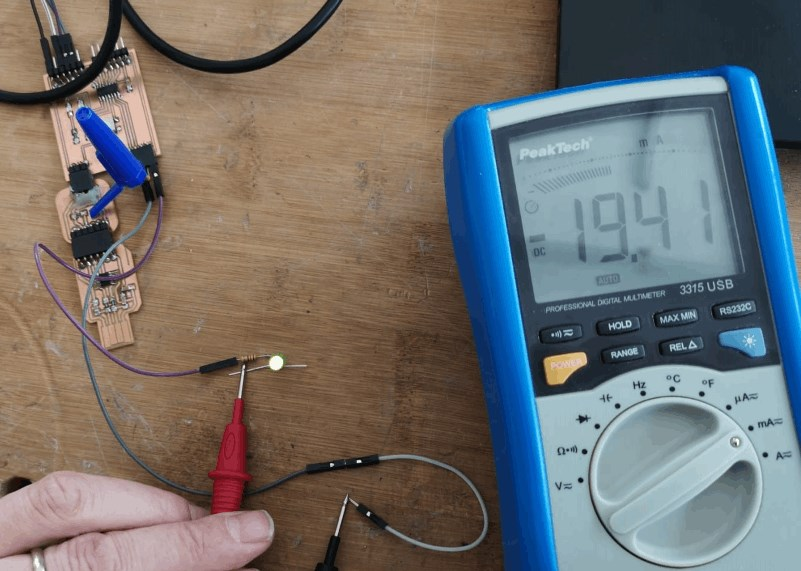Output Devices Group Assignment

The group assignment is to determine the power consumption of an output device, in my case for the final project I will be using DC motors so I am going to determine the power consumption of a DC motor.

The motor I am using is this one, and it has the following specifications:

• Gear Ratio: 107.15:1
• Max power: 2.2 W
• No-load speed @ 12V: 130 rpm
• No-load current @ 12 V: 0.08 A
• Stall Current @ 12 V: 1.6 A
• Torque hutch @ 12V: 6.5 kg.cm (90 oz.in)

These values are the values provided by the manufacturer which I am going to compare with the values measured by me.

To make the tests I connected the motor to a DC power supply at 12V, when activating the output of the power supply the motor starts to rotate at a constant speed, but if the voltage is decreased the rotation speed decreases, in the opposite case if the voltage is increased the speed increases. The demonstration is in the following video.

It can be seen that the motor without any load uses a current of 0.033A at 12V. To determine the consumption we apply the formula Power = Voltage * Amps replacing the terms we obtain Power = 12 * 0.033 = 0.396 Watt.

Based on the motor specifications it can be seen that the current consumption of the unloaded motor is lower in the measurements, and that the energy consumption (of course) is lower than the maximum.

Output Devices Group Assignment

Power consumption measurement¶

Since we had to measure the power consumption of an output device for this assignment, besided checking the datasheet of it, I used a power supply and a multimeter for this task; I have done the rest with a 9V battery, since my final project is going to work with batteries.

Voltage measurement (V)

I applied the voltage according to datasheet. (It is a 12V DC-motor)

This is done in parallel to the device: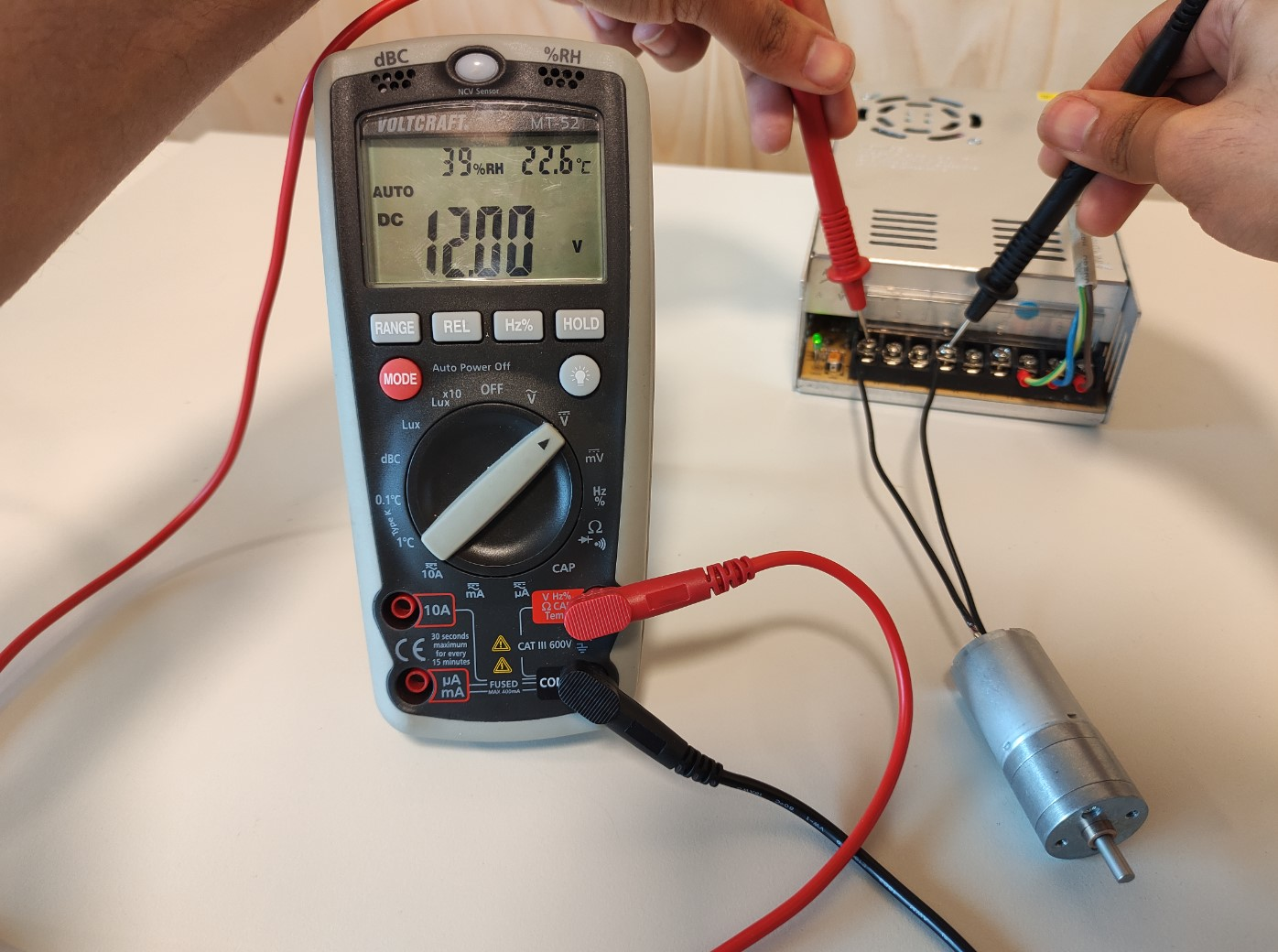Well, the result are the 12V applied. 🤷🏽‍♂️

Current measurement (I)

This is done in series to the device: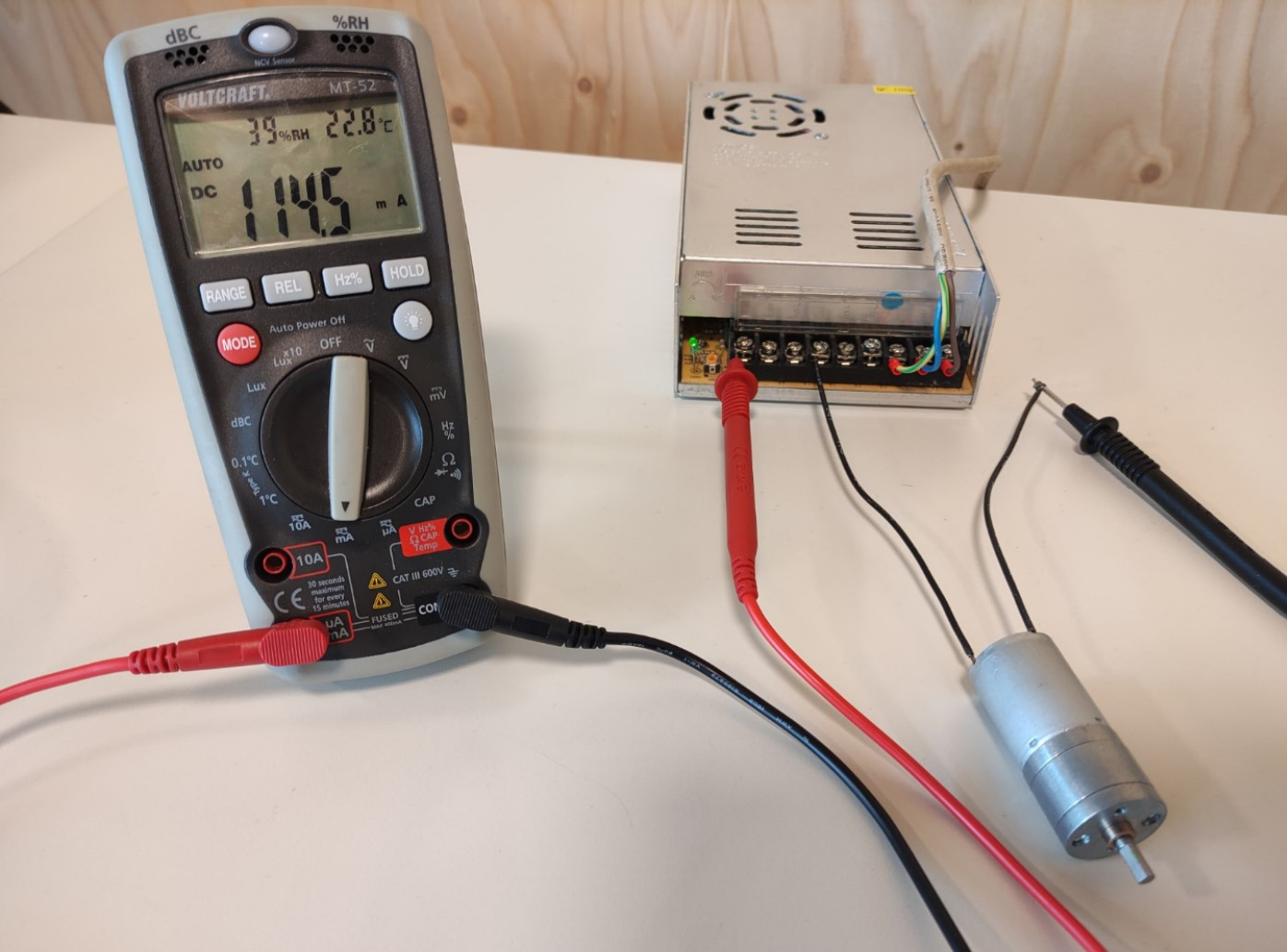Result: 114.5mA.

Power consumption (P) = V*I = 12V * 114.5mA = 1.37W

Datasheet information

In addition, I compared the values from the datasheet of the motor (a JGA25-370):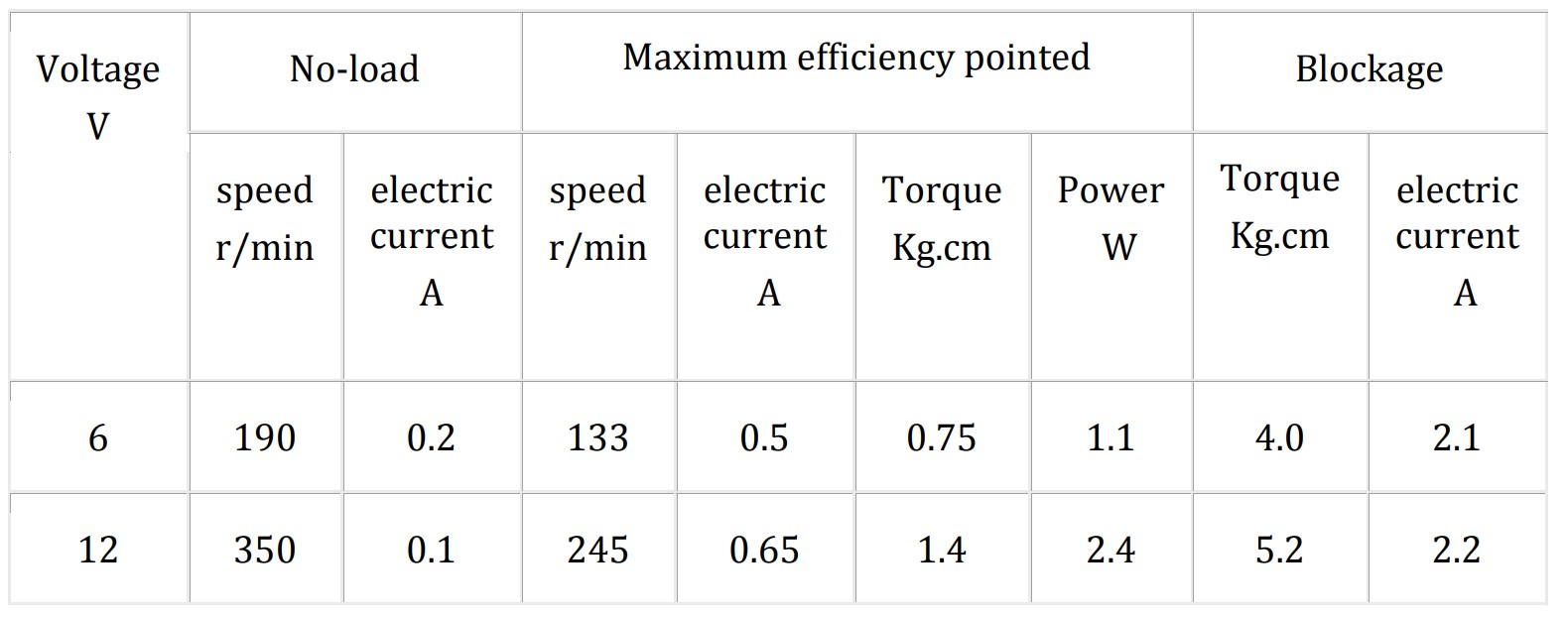The measured No-load electric current is 14.5mA higher than that from the datasheet.

Output Devices Group Assignment

In this example you can see very nicely how the consumption of the amperage increases when you put the motor under load by braking it with your thumb.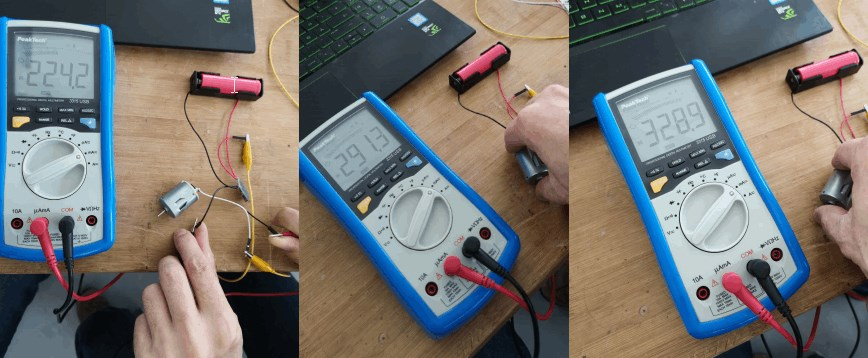Here we measure the current that is required by the LED to light up. In this case 19.41 mA.